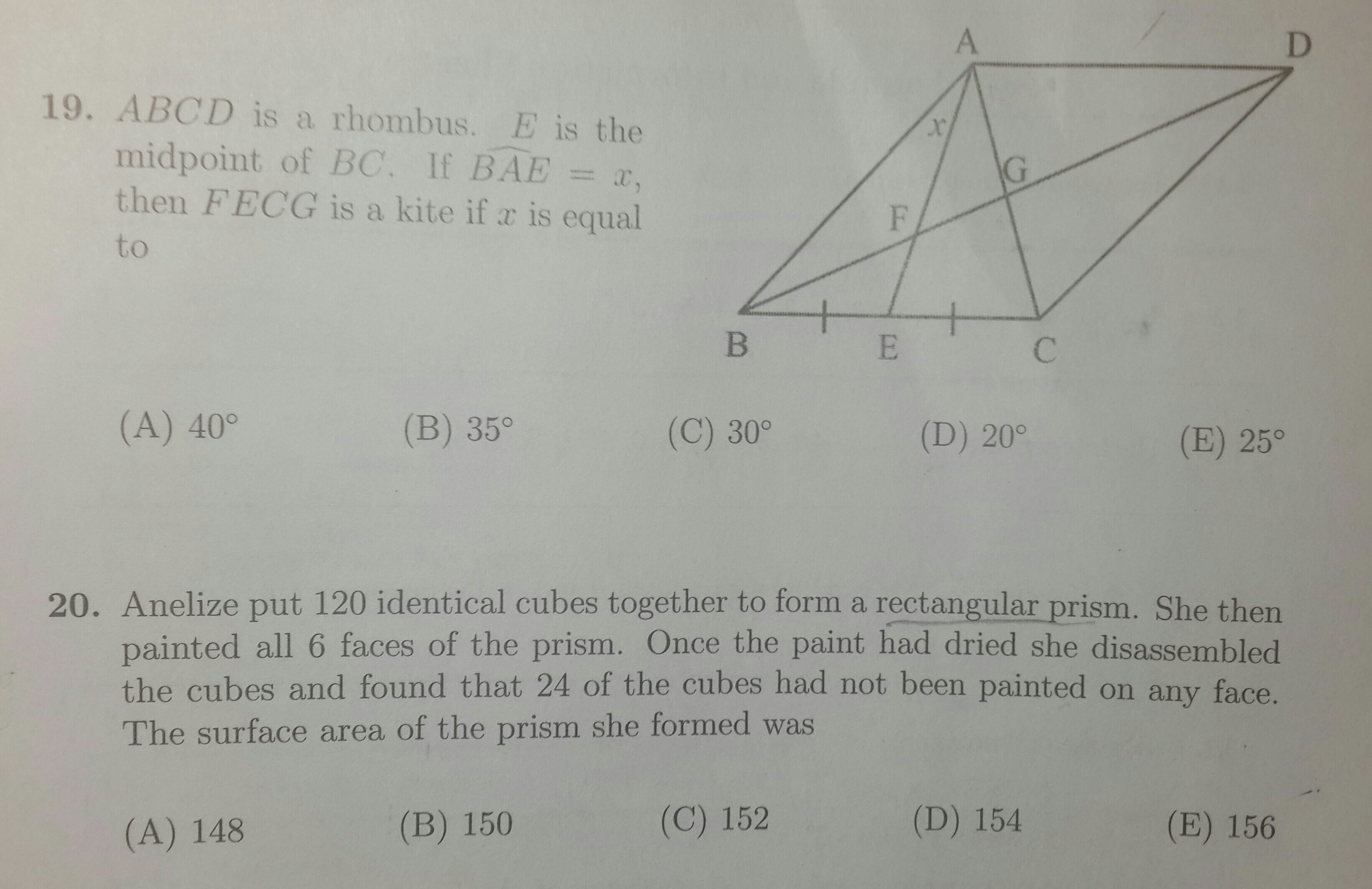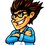# SA Mathematics Olympiad (2015) - 3 Tough QuestionsThe first round of the South African Mathematics Olympiad was held today at various high schools throughout the country. Can you solve the two hardest questions in the paper?Note by Mark Mottian
6 years, 4 months ago

This discussion board is a place to discuss our Daily Challenges and the math and science related to those challenges. Explanations are more than just a solution — they should explain the steps and thinking strategies that you used to obtain the solution. Comments should further the discussion of math and science.

When posting on Brilliant:

• Use the emojis to react to an explanation, whether you're congratulating a job well done , or just really confused .
• Ask specific questions about the challenge or the steps in somebody's explanation. Well-posed questions can add a lot to the discussion, but posting "I don't understand!" doesn't help anyone.
• Try to contribute something new to the discussion, whether it is an extension, generalization or other idea related to the challenge.

MarkdownAppears as
*italics* or _italics_ italics
**bold** or __bold__ bold
- bulleted- list
• bulleted
• list
1. numbered2. list
1. numbered
2. list
Note: you must add a full line of space before and after lists for them to show up correctly
paragraph 1paragraph 2

paragraph 1

paragraph 2

[example link](https://brilliant.org)example link
> This is a quote
This is a quote
    # I indented these lines
# 4 spaces, and now they show
# up as a code block.

print "hello world"
# I indented these lines
# 4 spaces, and now they show
# up as a code block.

print "hello world"
MathAppears as
Remember to wrap math in $$ ... $$ or $ ... $ to ensure proper formatting.
2 \times 3 $2 \times 3$
2^{34} $2^{34}$
a_{i-1} $a_{i-1}$
\frac{2}{3} $\frac{2}{3}$
\sqrt{2} $\sqrt{2}$
\sum_{i=1}^3 $\sum_{i=1}^3$
\sin \theta $\sin \theta$
\boxed{123} $\boxed{123}$

Sort by:

Solution to QUESTION 19 : ( Its not very complicated)

Let FECG be a kite , then

$FE=FG and EC=GC$

$GC=GA$ (Since diagonals of a rhombus bisect each other)

$EC=BE$ (Since $E$ is mid-point of $BS$

Therefore, $BC=AC$

But, $AB=BC$ (Sides of a rhombus)

So, $AB=BC=AC$

$\bigtriangleup ABC$ is an equilateral triangle

Since medians of an equilateral triangle also bisect the angle from which they are drawn,

$2x = 60$

$x=\boxed{30}$

- 6 years, 4 months ago

A really good solution! Good job!

- 6 years, 4 months ago

This challenging question also featured in the paper:

Triangle ABC has a point D on line AB such that D is the midpoint of AB. E is the midpoint of CD. F is the midpoint of AE. If the area of triangle ABC is 24, find the area of triangle DEF.

(A)   3
(B)   4
(C)   6
(D)   8
(E)   9


- 6 years, 4 months ago

Repeatedly using the fact that the midpoint of a triangle divides the triangle into $2$ triangles of equal area should get you the answer.

Hijacking @@Krishna Sharma's diagram, we have $[ADC] = \frac{1}{2}[ABC]$, $[ADE] = \frac{1}{2}[ADC]$ and $[DEF] = \frac{1}{2}[ADE]$. Combining all the equations, we have $[DEF] = \frac{1}{8}[ABC] = 3$

- 6 years, 4 months ago

Hi Siddhartha! This is a very insightful solution. If you knew that the "median of the triangle divides the triangle into two triangles of equal area", it makes the problem very easy. Your solution is definitely elegant. Thank you so much for sharing!

- 6 years, 4 months ago

yupp.. nice and easy.. without complications

- 6 years, 4 months ago

The answer is 3. Since nothing is given for simplicity consider the triangle is equilateral,find out the the square of side(yes side length not required), Now agian for simplicity consider the AB as base of triangle with vertex A at origin and we are done just find out coordinates of D,E,F in terms of 'a' and you are done!image

Sorry for my poor handwriting :p

- 6 years, 4 months ago

For 20, I don't see any easier solution other than seeing that a rectangular prism with side lengths $6,5,4$ works and thus the surface area is $148$.

- 6 years, 4 months ago

Siddhartha, how did you figure out that the side lengths were 4,5 and 6?

- 6 years, 4 months ago

Pretty much a lucky guess. We see that its the only solution to $xyz = 120$ and $(x-2)(y-2)(z-2) = 24$.

The first equation is obvious. The second one comes is from the fact that the total volume of the cuboid not colored is $(x-2)(y-2)(z-2)$, since we have to discount the cubes which appear on either ends.

- 6 years, 4 months ago

I think the answer of Q.19 is c) 30°.

- 6 years, 4 months ago